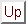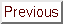Next: Multivariate Lagrange Interpolation Up: An introduction to the Previous: The basic trust-region algorithm   Contents

# About the CONDOR algorithm.

To start the unconstrained version of the CONDOR algorithm, we will basically need:
• A starting point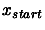• A lengthwhich represents, basically, the initial distance between the points where the objective function will be sampled.
• A length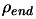which represents, basically, the final distance between the interpolation points when the algorithm stops.
DEFINITION: The local approximation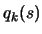ofis valid in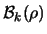(a ball of radiusaround) when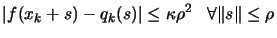where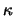is a given constant independent of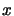.
We will approximatively use the following algorithm (for a complete description, see chapter 6):
1. Create an interpolation polynomialof degree 2 which intercepts the objective function around. All the points in the interpolation set(used to build) are separated by a distance of approximatively. Set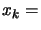the best point of the objective function known so far. Set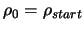. In the following algorithm,is the quadratical approximation ofaround, built by interpolation using(see chapter 3).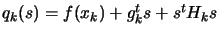where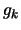is the approximate gradient ofevaluated atandis the approximate Hessian matrix ofevaluated at.
2. Set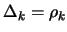3. Inner loop: solve the problem for a given precision of.
1. Solvesubject to.
2. If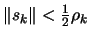, then break and go to step 3(b) because, in order to do such a small step, we need to be sure that the model is valid.
3. Evaluate the functionat the new position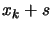. Update the trust region radius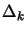and the current best pointusing classical trust region technique (following a scheme similar to Equation 2.20). Include the newinside the interpolation set. Updateto interpolate on the new.
4. If some progress has been achieved (for example,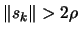or there was a reduction), increment k and return to step i, otherwise continue.
1. Test the validity of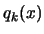in, like described in chapter 3.
• Model is invalid:
Improve the quality of the model: Remove the worst point of the interpolation setand replace it (one evaluation required!) with a new pointsuch that: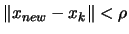and the precision ofis substantially increased.
• Model is valid:
Ifgo back to step 3(a), otherwise continue.
4. Reducesince the optimization steps are becoming very small, the accuracy needs to be raised.
5. If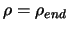stop, otherwise increment k and go back to step 2.
From this description, we can say thatandare two trust region radius (global and local).
Basically,is the distance (Euclidian distance) which separates the points where the function is sampled. When the iterations are unsuccessful, the trust region radiusdecreases, preventing the algorithm to achieve more progress. At this point, loop 3(a)i to 3(a)iv is exited and a function evaluation is required to increase the quality of the model (step 3(b)). When the algorithm comes close to an optimum, the step size becomes small. Thus, the inner loop (steps 3(a)i. to 3(a)iv.) is usually exited from step 3(a)ii, allowing to skip step 3(b) (hoping the model is valid), and directly reducingin step 4.
The most inner loop (steps 3(a)i. to 3(a)iv.) tries to get fromgood search directions without doing any evaluation to maintain the quality of(The evaluations that are performed on step 3(a)i) have another goal). Only inside step 3(b), evaluations are performed to increase this quality (called a ''model step'') and only at the condition that the model has been proven to be invalid (to spare evaluations!).
Notice the update mechanism ofin step 4. This update occurs only when the model has been validated in the trust region(when the loop 3(a) to 3(b) is exited). The function cannot be sampled at point too close to the current pointwithout being assured that the model is valid in.
The different evaluations ofare used to:
(a)
guide the search to the minimum of(see inner loop in the steps 3(a)i. to 3(a)iv.). To guide the search, the information gathered until now and available inis exploited.
(b)
increase the quality of the approximator(see step 3(b)). To avoid the degeneration of, the search space needs to be additionally explored.
(a) and (b) are antagonist objectives like usually the case in the exploitation/exploration paradigm. The main idea of the parallelization of the algorithm is to perform the exploration on distributed CPU's. Consequently, the algorithm will have better modelsofat disposal and choose better search direction, leading to a faster convergence.
CONDOR falls inside the class of algorithm which are proven to be globally convergent to a local (maybe global) optimum [CST97,CGT00b].
In the next chapters, we will see more precisely:
• Chapter 3: How to construct and use? Inside the CONDOR algorithm we need a polynomial approximation of the objective function. How do we build it? How do we use and validate it?
• Chapter 4: How to solve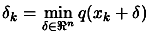subject to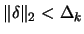? We need to know how to solve this problem because we encounter it at step (3)(a)i. of the CONDOR algorithm.
• Chapter 5: How to solve approximately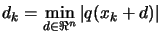subject to? We need to know how to solve this problem because we encounter it when we want to check the validity (the precision) of the polynomial approximation.
• Chapter 6: The precise description of the CONDOR unconstrained algorithm.Next: Multivariate Lagrange Interpolation Up: An introduction to the Previous: The basic trust-region algorithm   Contents
Frank Vanden Berghen 2004-04-19